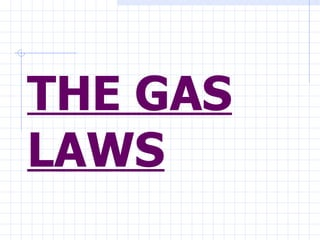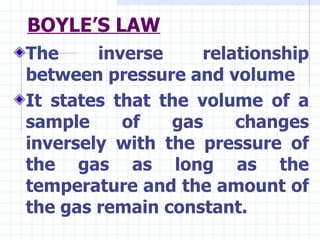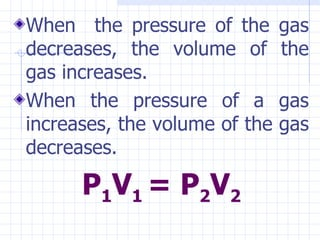Seu SlideShare está sendo baixado. ×

# The gas laws complete

Anúncio
Anúncio
Anúncio
Anúncio
Anúncio
Anúncio
Anúncio
Anúncio
Anúncio
Anúncio
AnúncioCarregando em…3
×

1 de 51 Anúncio

# The gas laws complete

Gas Laws

Gas Laws

Anúncio
Anúncio

### The gas laws complete

1. 1. THE GAS LAWS
2. 2. BOYLE’S LAW <ul><li>The inverse relationship between pressure and volume </li></ul><ul><li>It states that the volume of a sample of gas changes inversely with the pressure of the gas as long as the temperature and the amount of the gas remain constant. </li></ul>
3. 3. <ul><li>When the pressure of the gas decreases, the volume of the gas increases. </li></ul><ul><li>When the pressure of a gas increases, the volume of the gas decreases. </li></ul><ul><li>P 1 V 1 = P 2 V 2 </li></ul>
4. 4. <ul><li>SAMPLE PROBLEMS: </li></ul><ul><li>1. A tank of nitrogen has a volume of 14.0 L and a pressure of 760.0 mmHg. Find the volume of the nitrogen when its pressure is changed to 400.0 mmHg while the temperature is held constant. </li></ul>
5. 5. <ul><li>2. A 200-L helium gas at a pressure of 28 0 C and a pressure of 2.0 atm was transferred to a tank with a volume of 68.0 L. What is the internal pressure of the tank if the temperature is maintained? </li></ul>
6. 6. <ul><li>3. A balloon contains 14.0 L of air at a pressure of 760 torr. What will the volume of the air be when the balloon is taken to a depth of 10 ft in a swimming pool, where the pressure is 981 torr? The temperature of the air does not change. </li></ul>
7. 7. CHARLES’ LAW <ul><li>States that the Kelvin temperature and the volume of a gas are directly related when there is no change in pressure or amount of gas. </li></ul><ul><li>The relationship between volume and temperature was first observed by French physicist and balloonist Jacques Charles. </li></ul>
8. 9. SAMPLE PROBLEMS <ul><li>1. A balloon has a volume of 2500.0 mL on a day when the temperature is 30.0 0 C. If the temperature at night falls to 10.0 0 C, what will the volume of the balloon if the pressure remains constant? </li></ul>
9. 10. <ul><li>2. Find the final temperature of a 2.00- L gas sample at 20.0 0 C cooled until it occupies a volume of 500 mL. </li></ul>
10. 11. Do the ff. exercises: <ul><li>1. A sample of freon gas used in air conditioner has a volume of 325.0 L and a pressure of 96.3 kPa at 20 0 C. What will the pressure of the gas be when its volume is 975.0 L at 20 0 C? </li></ul>
11. 12. <ul><li>2. A balloon contains 30.0L of helium gas at 100 kPa. What is the volume when the balloon rises to an altitude where the pressure is only 25.0 kPa? </li></ul>
12. 13. <ul><li>3. What is the volume of a sample of thane at 467K and 2.25 atm if it occupies 1.405 L at 300K and 2.25 atm? </li></ul>
13. 14. <ul><li>4. The gas in a 1.00-L bottle at 25 0 C can be put into a 0.946 bottle at the same pressure if the temperature is reduced. What temperature is required? </li></ul>
14. 15. THE COMBINED GAS LAW <ul><li>The two gas laws can be combined and treated as a single law which describes the relationship among the pressure, volume and temperature of a constant amount of gas. </li></ul>
15. 16. SAMPLE PROBLEMS: <ul><li>1. A given mass of gas has a volume of 800.0 mL at –23.0 0 C and 300.0 torr. What would the volume of the gas be at 27.0 0 C and 600.0 torr of pressure? The amount of gas is constant. </li></ul>
16. 17. <ul><li>2. A 5.0 –L air sample at a temperature of –50.0 0 C has a pressure of 107.0 kPa. What will the new pressure be if the temperature is raised to 100.0 0 C and the volume expands to 7.0 L? </li></ul>
17. 18. <ul><li>3. A 3.50-L gas sample at 20 0 C 20 0 C and a pressure of 86.7 kPa is allowed to expand to a volume of 8.0 L. The final pressure of the gas is 56.7 kPa. What is the final temperature of the gas? </li></ul>
18. 19. <ul><li>5. A weather balloon is filled with 16.0 L of helium at a temperature of 26 0 C and a pressure of 700 mm hg. What is the pressure of the helium in the balloon in the upper atmosphere when the temperature is -33 0 C and the volume becomes 35.oL </li></ul>
19. 20. THE IDEAL GAS LAW <ul><li>The law that describes the pressure, volume, temperature, and number of moles of a gas. </li></ul>
20. 21. <ul><li>PV= nRT </li></ul><ul><li>Where: </li></ul><ul><li>P= pressure of the gas </li></ul><ul><li>V= volume of the gas </li></ul><ul><li>n= amount of gas </li></ul><ul><li>R= ideal gas constant </li></ul><ul><li>T= temperature of the gas </li></ul>
21. 22. <ul><li>At STP, </li></ul><ul><li>P= 1.0 atm= 101.325 kPa </li></ul><ul><li>V= 22.4 L </li></ul><ul><li>n= 1.0 mole </li></ul><ul><li>T= 273 K </li></ul><ul><li>R= 0.0821 atm x L/ mol x K </li></ul><ul><li> = 8.31kPa x L/ mol x K </li></ul>
22. 23. SAMPLE PROBLEMS: <ul><li>1. What volume will 1.27 mol of helium gas occupy at STP? </li></ul>
23. 24. <ul><li>2. How many moles of gas are contained in a 50.0-L cylinder at a pressure of 100.0 atm and a temperature of 35.0 0 C? </li></ul>
24. 25. <ul><li>3. What pressure will be exerted by 0.450 mol of a gas at 25 0 C if it is contained in a vessel whose volume is 0.65 L? </li></ul>
25. 26. 4. You fill a rigid steel cylinder with a volume of 20.0 L with nitrogen gas to a final pressure of 20 000 kPa at 27 0 C. How many moles of nitrogen gas does the cylinder contain?
26. 27. Answer the following exercises: <ul><li>1. A deep underground cavern contains 2.24 x 10 6 L of methane gas(CH 4 ) at a pressure of 1,500 kPa and a temperature 42 0 C. How many kg of methane does this gas deposit contain? </li></ul>
27. 28. <ul><li>2. The volume of a gas- filled balloon is 30.0 L at 40.0 0 C and 150 kPa pressure. What volume will the balloon have at STP? </li></ul>
28. 29. <ul><li>3. The volume of a sample of CO is 405 mL at 10.0 atm and 467 K. What volume will it occupy at 4.29 atm and 467 K? </li></ul>
29. 30. <ul><li>4. When a rigid hollow sphere containing 680 L of helium gas is heated from 300 K to 600 K, the pressure of the gas increases to 1 800 kPa. How many moles of helium are in the sphere? </li></ul>
30. 31. <ul><li>5. A container with an initial volume of 1.0 L is occupied by a gas at a pressure of 150 kPa at 25 0 C. By changing the volume, the pressure of the gas increases to 600 kPa as the temperature is raised to 100 0 C. What is the new volume? </li></ul>
31. 32. GAY- LUSSAC’S LAW <ul><li>Temperature and pressure relationship </li></ul><ul><li>The pressure of a gas is directly related to its Kelvin temperature. </li></ul>
32. 33. <ul><li>This means that an increase in temperature increases the pressure of a gas, and a decrease in temperature decreases the pressure of the gas as long as the volume and number of moles of the gas remain constant. </li></ul>
33. 35. SAMPLE PROBLEMS: <ul><li>1. The gas left in a used aerosol can is at a pressure of 100 kPa at 27 0 C. If the can is thrown onto a fire, what will the internal pressure be when its temperature reaches 927 0 C? </li></ul>
34. 36. <ul><li>2 . The pressure in automobile tire is 200 kPa at a temperature of 27 0 C. At the end of a journey on a hot sunny day, the pressure has risen to 223 kPa. What is the temperature of the air in the tire? </li></ul>
35. 37. AVOGADRO’S LAW <ul><li>Equal volumes of gases at the same temperature and pressure contain equal numbers of particles. </li></ul>
36. 39. SAMPLE PROBLEMS <ul><li>If 23.1 g of oxygen occupies a volume of 5.0 L at a particular pressure and temperature, what volume will 2.5 g of oxygen gas occupy under the same conditions? </li></ul>
37. 40. <ul><li>Determine the volume in liters occupied by 0.202 mol of a gas at STP. </li></ul><ul><li>How many oxygen molecules are in 3.3 liters of oxygen gas at STP? </li></ul>
38. 41. <ul><li>Determine the volume in liters occupied by 14.0 g of N 2 at STP. </li></ul><ul><li>What volume is occupied by 4.02 x 10 22 molecules of H 2 at STP? </li></ul>
39. 42. DALTON’S LAW <ul><li>At constant volume and temperature, the total pressure exerted by a mixture of gases is equal to the sum of the partial pressures of the component gases. </li></ul>
40. 43. <ul><li>P total = P 1 +P 2 +P 3 …… </li></ul>
41. 44. SAMPLE PROBLEMS <ul><li>Determine the total pressure of a gas mixture that contains oxygen, nitrogen, and helium if the partial pressures of the gases are 20.0 kPa, 46.7 kPa, and 26.7 kPa respectively. </li></ul>
42. 45. <ul><li>A balloon contains mostly He and a little methane. The partial pressure of helium is 101.2 kPa. If the pressure inside the balloon is 101.3 kPa, what is the partial pressure of methane? </li></ul><ul><li>A 5.73- L flask at 25 0 C contains 0.0388 mol of N 2 , 0.1 47 mol of CO, and 0.0803 mol of H 2 . What is the pressure in the flask in atm and kPa? </li></ul>